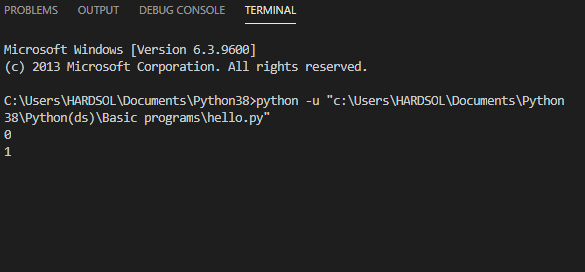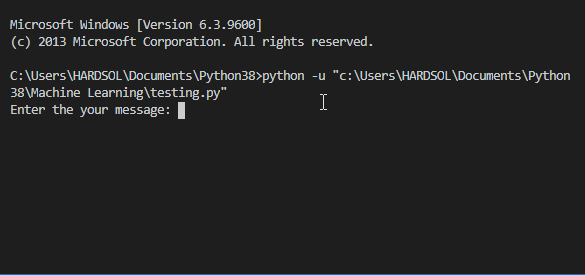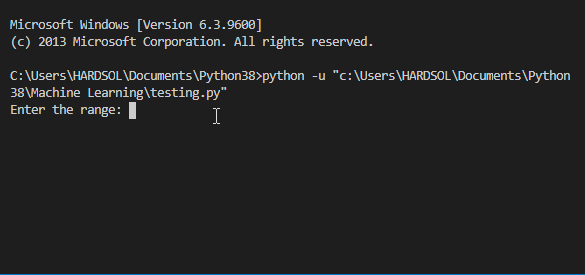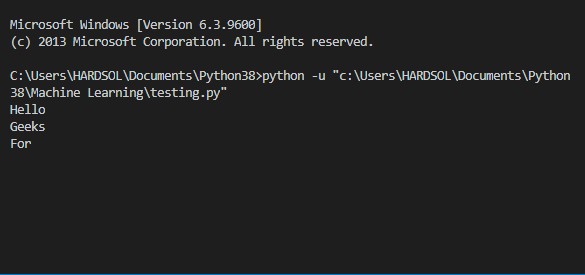# How to add time delay in Python?

• Difficulty Level : Hard
• Last Updated : 24 Jan, 2021

In this article, we are going to discuss how do we introduce or add time delay in our program code.

Time Delay

Attention geek! Strengthen your foundations with the Python Programming Foundation Course and learn the basics.

To begin with, your interview preparations Enhance your Data Structures concepts with the Python DS Course. And to begin with your Machine Learning Journey, join the Machine Learning - Basic Level Course

• In order to add time delay in our program code, we use the sleep() function from the time module. This is the in-built module in Python we don’t need to install externally.
• Time delay means we are adding delay during the execution time in our program code. It should be between two statements or between any part of the program code according to you.

Syntax:

`time.sleep(value)`

Approach:

• Import the time module
• For adding time delay during execution we use the sleep() function between the two statements between which we want the delay. In the sleep() function passing the parameter as an integer or float value.
• Run the program.
• Notice the delay in the execution time.

To understand the topic perfectly. Let’s see the implementation by taking some examples.

Note: As an output, I have shown the GIF, so that you can notice the time delay in program code during the execution time.

Example 1: Printing the numbers by adding a time delay.

## Python3

 `# importing module``import` `time`` ` ` ` `# running loop from 0 to 4``for` `i ``in` `range``(``0``,``5``):``   ` `  ``# printing numbers``  ``print``(i)``   ` `  ``# adding 2 seconds time delay``  ``time.sleep(``2``)`

Output:Example 2: Dramatic printing using sleep() for every character.

## Python3

 `# importing time module``import` `time`` ` ` ` `def` `message(string):``   ` `    ``for` `i ``in` `string:``       ` `        ``# printing each character of the message``        ``print``(i, end``=``"")``         ` `        ``# adding time delay of half second``        ``time.sleep(``0.5``)`` ` ` ` `# main function``if` `__name__ ``=``=` `'__main__'``:``    ``msg ``=` `"Its looks like auto typing"``     ` `    ``# calling the function for printing the ``    ``# characters with delay``    ``message(msg)`

Output:Example 3: Printing the pattern by taking range from the user and adding time delay.

## Python3

 `# importing module``import` `time`` ` ` ` `# function to print the pattern``def` `pattern(n):``   ` `    ``for` `i ``in` `range``(``0``, n):``        ``for` `j ``in` `range``(``0``, i``+``1``):``           ` `            ``print``(``'*'``, end``=``' '``)``             ` `            ``# adding two second of time delay``            ``time.sleep(``0.5``)``        ``print``(``' '``)`` ` ` ` `# main function``if` `__name__ ``=``=` `'__main__'``:``   ` `    ``# taking range from the user``    ``num ``=` `4``    ``print``(``"Printing the pattern"``)``     ` `    ``# calling function to print the pattern``    ``pattern(num)`

Output:## Python3

 `# importing``import` `time``from` `threading ``import` `Thread`` ` ` ` `# making first thread of Geeks``class` `Geeks(Thread):``   ` `    ``def` `run(``self``):``        ``for` `x ``in` `range``(``4``):``            ``print``(``"Geeks"``)``             ` `            ``# adding delay of 2.2 seconds``            ``time.sleep(``2.2``)`` ` `# making second thread of For``class` `For(Thread):``   ` `    ``def` `run(``self``):``        ``for` `x ``in` `range``(``3``):``            ``print``(``'For'``)``             ` `            ``# adding delay of 2.3 seconds``            ``time.sleep(``2.3``)`` ` ` ` `print``(``"Hello"``)`` ` `# making the object for both the ``# threads separately``g1 ``=` `Geeks()``f1 ``=` `For()`` ` `# starting the first thread``g1.start()`` ` `# starting the second thread``f1.start()`` ` `# waiting for the both thread to join``# after completing their job``g1.join()``f1.join()`` ` `# when threads complete their jobs``# message will be printed``print``(``"All Done!!"``)`

Output:My Personal Notes arrow_drop_up